# Convert Text To Numbers In ExcelArticle byExcelMojo Team## Convert Text To Numbers In Excel

Convert text to numbers in Excel is the process that helps convert numbers, having Text data format, into numeric values. Users can thus perform numeric calculations and arithmetic operations on the converted numbers.

The feature is useful to check if the numbers in the spreadsheet are not in the Text format, thus ensuring you can use them in mathematical evaluations error-free. For example, the below table contains a list of text strings and values that appear as numeric data but are texts.

Suppose the requirement is to convert the given texts into numbers and display the result in column B. Then, the following formulas can help us achieve the required outcome.

The column B formulas return numeric values. While some use Excel functions, such as VALUE(), others use mathematical equations to get the result.

Note that the cell alignment for text values in column A is left. On the other hand, the column B cells show a right alignment, indicating their content is numeric, and column C contains the column B cells’ data format information.

###### Key Takeaways
• The convert text to numbers in Excel technique helps convert text formatted numbers into numbers.
• Users can utilize this option to ensure the numeric values they aim to use in mathematical evaluations are numbers, not texts.
• We can identify if a number has a text format by checking if the value has a left alignment. And the Status Bar does not show the sum and average value on selecting the entire data range. On the other hand, the Formula Bar will show a leading apostrophe preceding the value, and thus the cell shows the error indicator.
• We can use the error handle box, Paste Special feature, Text to Columns wizard, VALUE(), or mathematical operations to convert text to numbers in Excel.

### How To Identify Numbers Formatted As Text In Excel

To convert text to numbers in Excel, we can use the following ways to identify numbers formatted as text.

Let us see them with an example.

The following table contains a list of texts that appear as numeric values and their respective cell data format or type.

#### Check The Error Indicator

Cells A2 and A4 show a green triangle in the top-left corner. It is an error indicator. And when we click such a cell, we will see a warning symbol that we can click to check the error.

In this case, as the data stored is a number, but with an apostrophe in the front, Excel does not consider it as a numeric value. And the error message confirms the same.

#### Check The Cell Value Visually

A cell containing a number will have the right alignment as the default alignment. Also, if we select multiple cells containing numbers, we will get values such as sum and average in the Status Bar in Excel.

For example, column A cells have a left alignment, indicating their content is text. Also, when we select the column A data, the Status Bar does not show the sum and average.

And while cell data, such as in cell A2, will have an apostrophe preceding it, some have the data format in HomeNumber Format set as Text, such as cell A4.

But suppose we convert the column A texts into numbers using the VALUE Excel function and display the numeric values in column C in the above table.

Then, the column C data shows the resulting numeric values have a right alignment, with the values without an apostrophe in the front.

And on selecting column C numeric data, the Status Bar shows the average and sum values. Further, if we check the Home -> Number Format settings for the column C cells in question, it will not be Text. Instead, their data type will be as mentioned in column D, all indicating column C values as numeric.

### Top 5 Methods To Convert Text To Numbers In Excel

The following five methods will help us convert text to numbers in Excel.

#### #1 – Using Quick Convert Text To Numbers Excel Option

This example shows the shortcut to convert text to numbers in Excel.

The below table shows a list of students and their Mathematics scores.

However, the cells containing the scores show the error indicator. And on checking the error using the error handle box, it shows the scores are texts and not numbers. The reason is that the numbers have an apostrophe in the front.

In such a case, we can apply the shortcut to convert text to numbers in Excel, the error-checking method.

• Step 1: Select the cell range C2:C11.
• Step 2: Click the error handle box and pick the second option to convert text to numbers in Excel cell range C2:C11.

#### #2 – Using Paste Special Cell Formatting Method

The following table contains the half-yearly income data of a professional.

However, the cell range B2:B7 containing the monthly income figures show an error. Checking the error using the error handle box shows that the income values are texts, not numbers. And on summing this cell range values in cell C8, we get the total half-yearly income as \$0.

Thus, the solution is to use the Paste Special feature to convert text to numbers in Excel cells B2:B7. And the steps are as follows:

• Step 1: We shall introduce a new cell D2 containing the number value of 1.
• Step 2: Copy cell D2 by right-clicking the cell and selecting Copy from the contextual menu or pressing the Ctrl + C keys.
• Step 3: Select the cell range B2:B7, containing the monthly income values as text numbers. And press Alt + E + S + V to open the Paste Special Window and select the Paste option Values.

Next, pick the Operation setting, Multiply, and click OK to close the window and view the text values converted into numbers in cell range B2:B7.

The Paste Special feature multiplies cell D2 value, 1, with each monthly income value in the selected cell range B2:B7, thus converting them into numbers.

Further, as the cell range B2:B7 values become numbers, the cell B8 SUM Excel function adds all the monthly income figures and returns the correct total half-yearly income, \$14,500.

#### #3 – Using The Text To Column Method

The Data tab’s Text to Columns wizard enables one to convert text to numbers in Excel.

Let us see the steps with an example.

The table below shows the stock movers and their per day volume and percentage data as text strings in column B.

Suppose the requirement is to separate the volume and percentage figures and display them in columns C and D.

Then, here is how we can utilize the Text to Columns wizard from the Data tab and get the %CHG values as numbers from the column B text values.

• Step 1: First, copy the column B data into column C.
• Step 2: Select column C and click DataText to Columns to open the wizard window.

Set the Original data type as Delimited and click Next, as highlighted above.

• Step 3: Under Delimiters, check the Other check box, enter ‘_’ in the field against it, and click Finish.
• Step 4: As the above steps split the column C data, placing the data in the next column D, and column D contains a column heading, Excel will show the below warning message.

Click OK to view the below result.

• Step 5: Update cell D1 with the column heading to complete the table.

Thus, column D contains numbers we can use for future mathematical calculations.

#### #4 – Convert Text To Number Formula

The Excel function VALUE helps convert text to numbers in Excel.

The VALUE() takes one mandatory argument, text, as input. We can supply the text string we want to convert into the number it represents directly as the function argument. Otherwise, the function argument can be an Excel cell reference to the text string or a formula returning the text string.

For example, the below table contains a list of text strings.

Suppose the requirement is to convert the texts given in column A or extracted from the column A strings into numbers and display the result in column B. Then, here is how we can apply the VALUE() in the target cells and achieve the required data.

• Step 1: Select the target cell B2, enter the VALUE(), and press Enter.

=VALUE(A2)

The VALUE() accepts the date value given as a string in cell A2 and returns the serial number equivalent to the specified date, 44907. But as the data format set in Home -> Number Format is Short Date, we obtain the result as 12/12/2022.

• Step 2: Select the target cell B3, enter the VALUE(), and press Enter.

=VALUE(A3)

The VALUE() accepts the time value given as a string in cell A3 and returns the decimal equivalent of the specified time, 0.875. But as the data format set in Home -> Number Format is Time, we obtain the result as 9:00:00 PM.

• Step 3: Select the target cell B4, enter the VALUE(), and press Enter.

=VALUE(“\$5,000”)

The VALUE() accepts the cell A4 currency value as a string and returns the integer equivalent of the specified currency value, 5000. But as the data format set in Home -> Number Format is Currency, we obtain \$5,000.00 as the output.

• Step 4: Select the target cell B5, enter the VALUE(), and press Enter.

=VALUE(LEFT(A5,4))

The LEFT() accepts the cell A5 string and four characters to extract from the left of the given string as input. So, it returns the text 1345 as output, which the VALUE() takes as input to convert the text into the number 1345.

#### #5 – Turn Text Into Number With Mathematic Operations

Applying the appropriate mathematical operations can help convert text to numbers in Excel.

This method requires us to create formulas using arithmetic operators.

For example, the below table contains a list of numeric values in the text format.

Suppose the requirement is to convert the texts into numbers and display the results in column C. Then, here is how we can use mathematical operations in the target cells and achieve the required number values.

• Step 1: Select the target cell C2, enter the below formula, and press Enter.

=B2*1

The above expression multiplies the cell B2 value with 1 to return the value 3500. However, the data format of cell C2 set in HomeNumber Format is Currency. And thus, we get the output as \$3,500.

• Step 2: Select the target cell C3, enter the below formula, and press Enter.

=B3+0

The above expression adds 0 to the cell B3 value to return the sum as 0.6. However, the data format of cell C3 set in HomeNumber Format is Percentage. And thus, we get the output as 60.00%.

• Step 3: Select the target cell C4, enter the below formula, and press Enter.

=B4/1

The above expression divides the cell B4 value to return the quotient as 1938, a number with the data format in HomeNumber Format being General.

The above mathematical formulas do not change the data values. Instead, they convert the data format from text into numbers.

We can also utilize these mathematical operations to convert text to numbers in Excel VBA. And for that, we will have to use the respective arithmetic operators to form the expressions in the VBA code.

### Important Things To Note

• If a cell shows a green triangle in the top-left corner, click the cell to view the error handle box. And you will see a hover message saying the number inside the cell has a text format. You can then click the error handle box and choose the second option to convert text to numbers in Excel.
• When using the VALUE(), ensure the text argument is a text string, a cell reference to a string, or a formula returning a text string that you require to convert into numbers.
• We can enter mathematical expressions in cells to change the text to numbers or apply them to convert text to numbers in Excel VBA. But ensure they keep the cell values the same.

1. Is there a function to convert text to numbers in Excel?

There is a function to convert text to numbers in Excel, which is the VALUE().

It takes one mandatory argument, text, as input. And the text argument can be a text string we require to convert into numbers, a cell reference to a text string, or a formula returning a string.

2. How to convert text to numeric values in Excel VBA?

We can convert text to numeric values in Excel VBA using mathematical operations.

For example, the following table contains a list of numbers in text format.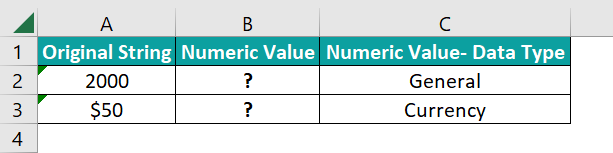Suppose the requirement is to convert the given text values into numeric values and display the result in column B. And column C shows the data format set in each column B cell in HomeNumber Format. Then, here is how we can use Excel VBA for the text to numbers conversion.

• Step 1: With the current worksheet containing the above table open, open the VBA Editor using the Alt + F11 keys.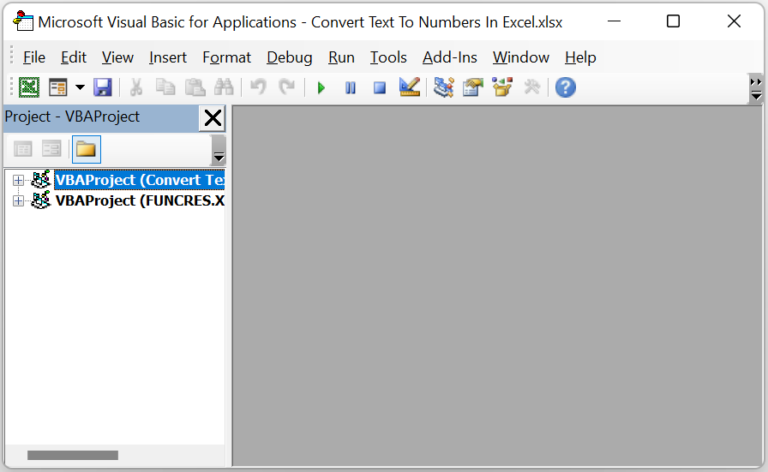• Step 2: Choose the applicable VBAProject and select Module from the Insert tab to open a module window.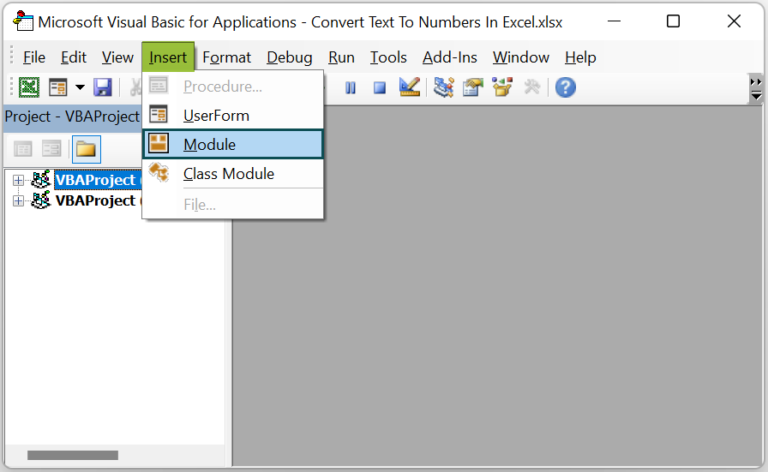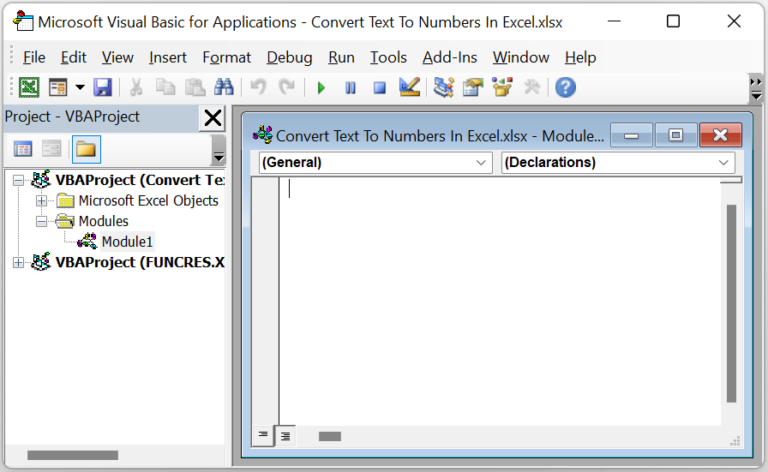• Step 3: Enter the VBA code in the module window to implement the required mathematical operations in the target cells.

Sub ConvertTN_fn()

Dim ws As Worksheet
Set ws = Worksheets(“ConvertTN_FAQ”)

ws.Range(“B2”) = ws.Range(“A2”) / 1
ws.Range(“B3”) = ws.Range(“A3”) * 1

End Sub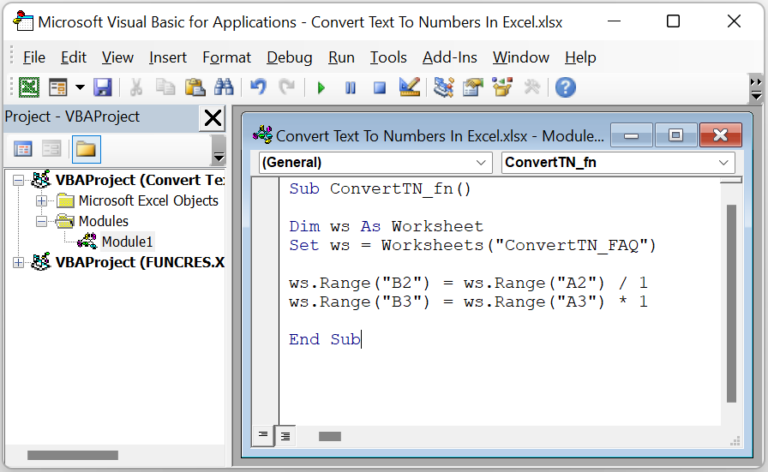• Step 4: Click the Run Sub/UserForm icon to execute the code.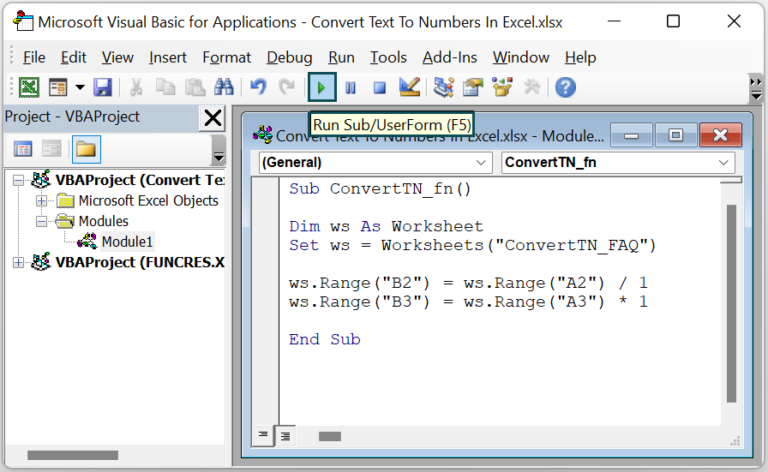We can now open the active worksheet to view the results in the target cells.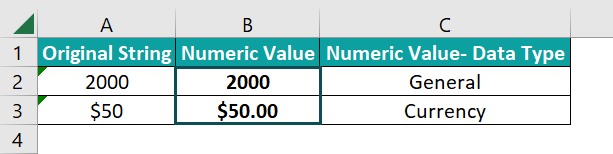While the applied mathematical operations in cell range B2:B3 convert the text strings into numbers, they do not alter the original values.

3. Why is converting text to numbers not working in Excel?

The converting text to numbers is not working in Excel, perhaps due to the following reasons:
While using the Paste Special feature, you multiply the text strings you require to convert into numbers with 0.
We chose incorrect delimiters while applying the Text to Columns wizard.
We supplied an incorrect text string, an invalid cell reference to a text string, or a formula not returning the correct text string as the VALUE() argument.
The applied mathematical operation is incorrect.